RocFall3 | Your new tool for 3D assessment of slopes at risk for rockfalls. Get started with RocFall3 today!

# Fisher Distribution

A Fisher Distribution is commonly used for modelling the distribution of 3-dimensional orientation vectors [Fisher (1953)], such as the distribution of joint orientations (pole vectors) on a sphere. A Fisher Distribution describes the angular distribution of orientations about a mean orientation vector and is symmetric about the mean. The probability density function can be expressed as: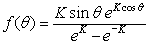Eqn.1

where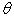is the angular deviation from the mean vector, in degrees, and K is the "Fisher constant" or dispersion factor.

In SWedge, a Fisher distribution can be used to define probabilistic joint orientation or tension crack orientation.

## Fisher K

The Fisher K value describes the tightness or dispersion of an orientation cluster. A larger K value (e.g. 50) implies a tighter cluster, and a smaller K value (e.g. 20) implies a more dispersed cluster, as shown in the following figure.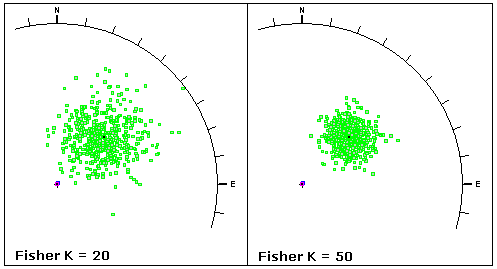Effect of Fisher K on a randomly generated joint set of 500 samples

The Fisher K value can be estimated from Eqn. 2, for data sets with greater than approximately 30 vectors (poles) [Fisher (1953)].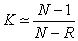Eqn.2

where N is the number of poles, and R is the magnitude of the resultant vector (i.e. the magnitude of the vector sum of all pole vectors in the set).

## Standard Deviation

By analogy with a Normal distribution, it can be shown that the standard deviation of a Fisher distribution can be estimated from Eqn.3 [Butler (1992)].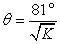Eqn.3

whereis the "angular standard deviation" or "angular dispersion" of the Fisher Distribution, and K is the Fisher constant.